# MOSFET (Si/SiC) Forum Discussions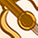Level 3Level 3

# IPA60R180P7 Coer, Cotr Questuon

Dear Sir,

Is there a detailed description of the physical meaning of Coer, Cotr?
How to use Coer, Cotr to evaluate turn-off loss?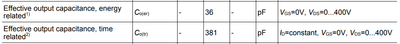1 SolutionModeratorModerator

# Re: IPA60R180P7 Coer, Cotr Questuon

Hi KUOWENYUNG，

Welcome to Infineon Developer Community，

In the diagram14 of the datasheet，typical capacitances as a function of the drain-source voltage are defined. We can see from the below figure，the value of Coss is not constant. The  Coss value listed in the datasheet is the value under a certain conditions，such as Coss = 19pF@VGS=0V，VDS=400V and f=250kHz.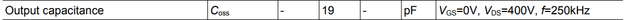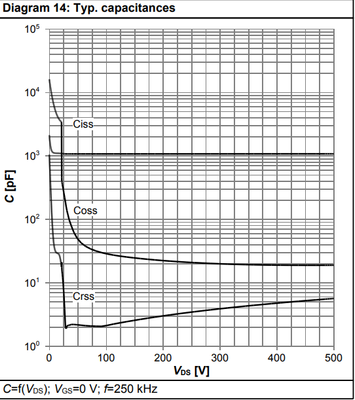So Coer and Cotr are defined and explained in details in the datasheet. Coer is a fixed capacitance that gives the same energy as Coss while Vds is rising from 0 to 400V. Cotr is a fixed capacitance that gives the same charging time as Coss while Vds is rising from 0 to 400V.

Cotr is used solely for calculating any resonant circuit that involves the output capacitance of the MOSFET.

Coer is usually used for calculating any hard switching power supply circuit that involves the output capacitance of the MOSFET.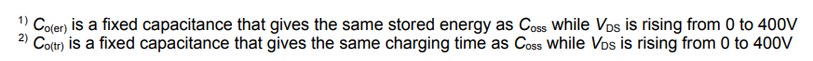You can refer to the following formula to calculate the Coss power loss：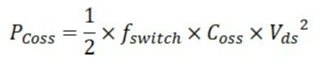Best regards，

RachelModeratorModerator

# Re: IPA60R180P7 Coer, Cotr Questuon

Hi KUOWENYUNG，

Welcome to Infineon Developer Community，

In the diagram14 of the datasheet，typical capacitances as a function of the drain-source voltage are defined. We can see from the below figure，the value of Coss is not constant. The  Coss value listed in the datasheet is the value under a certain conditions，such as Coss = 19pF@VGS=0V，VDS=400V and f=250kHz.So Coer and Cotr are defined and explained in details in the datasheet. Coer is a fixed capacitance that gives the same energy as Coss while Vds is rising from 0 to 400V. Cotr is a fixed capacitance that gives the same charging time as Coss while Vds is rising from 0 to 400V.

Cotr is used solely for calculating any resonant circuit that involves the output capacitance of the MOSFET.

Coer is usually used for calculating any hard switching power supply circuit that involves the output capacitance of the MOSFET.You can refer to the following formula to calculate the Coss power loss：Best regards，

Rachel